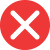gramatically

Incorrect spelling, explanation: gramatically is incorrect because the root noun grammar is spelled with double m, which is not changed in the case of later forms and suffixes. Therefore grammatically is the correct adverb. Grammatical is the adjective from which we make the adverb grammatically.grammatically

Correct spelling, explanation: this is the correct spelling because double m already occurs in Latin grammatica, from which we got the root word for grammatically – the noun grammar. Gramatically is therefore an incorrect spelling while the correct one is grammatically.

Definition of grammatically:
There are a lot of grammatically incorrect statements in your paper, please correct them.grammaticaly

Incorrect spellinggramaticaly

Incorrect spellinggramatticaly

Incorrect spelling